# Bias towards what we think is “truth” blinds us to fallacious arguments, not just to evidence that contradicts our beliefs.

Usually confirmation bias is associated with selecting factual evidence that confirms our pre-existing beliefs and rejecting evidence that contradicts them. However, there is another kind of confirmation bias, which I think is both more subtle and more dangerous than bias in selecting evidence. This is a confirmation bias towards fallacious arguments.

The danger lies not just in coming to the wrong conclusion in that one instance. The deeper danger is the following: Even if the conclusion in this one instance is true, we ourselves may repeat the fallacious argument in a different context and conclude something untrue. In general, it prevents us from learning valid argumentation and rational thinking, two of the foundations of science. Further, the truth of the one conclusion bolsters the reputation of the person making the argument even when the argument itself is fallacious and later used to reach incorrect conclusions, especially if we also have a penchant for believing authority.

This happens to scientists and mathematicians as well. When I have wanted something to be true, I have bought my own argument in favor of the conclusion too easily. Others have too easily conceded “Makes sense.” to an argument I’m still developing; this is made easier when the outcome is potentially beneficial to us — eking out more margin in Ad Tech e.g.. When I am in a position of “authority”, e.g. during my Stand-up Physics show to a range of audiences from elementary school kids to people at tech companies, if my “explanation” is plausible people buy it. So now I’ve changed the show: I do not explain anything we see — in fact I don’t even tell them what to look for — and I simply provide ways for the audience to test their own explanations or question those of others. When I was teaching college Physics, I had to make intentional mistakes in order to get the students to lose a bit of faith in me, think for themselves and not take my word for it.

Let me give you two examples -from Mathematics/Physics- of very convincing but fallacious arguments for things that are true. Feel free to poke holes.

# Random Walks

My college friends and I first came across random walks in the second year or so of engineering college. The simplest random walk is to take a single step of unit size every uniform time interval, the randomness lies in selecting a random direction for each step ‘t’. It looks something like this after a few steps: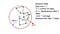Fig. Walk1: A random walk of unit step size starting from ‘O’. The dashed circles represent average displacements after ‘t’ (black) and ‘t+1’ (red) steps.

Of course, since it is random, you can’t predict where you are at step ‘t’ (other than “not greater than ‘t’ from the start”). But you can ask how far away you are from the start after ‘t’ steps d(t), on average<d(t)>. ‘d(t)’ is the distance from the origin after ‘t’ steps, and < > simply indicates that we are taking the average over many random walks of the same number of steps. It turns out that on average, you are

away from the start after ‘t’ steps. You can look up Wikipedia, random walks grow on average like the square-root of the number of steps.

To most of us, this was a puzzle, I don’t think we were given a good proof, or perhaps we didn’t understand it well enough to be able to reproduce it. But one of us, extremely knowledgeable about Math.s and Physics and #2 at our elite institution to boot, had a simple proof. (Now I would call it more of a plausibility argument than anything else.) It went something like this: Since the walk is random and goes in all possible directions at any step, the average area filled by the walk increases linearly in time. So <area(t)> ~t, but since the area within the average circle is the square of the average radius or distance from the center: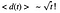Eq. Walk1

Convinced? We were. We all nodded our heads in awe, but none of us, as I recall, questioned the argument. Were we to have exercised our brains and extended the argument to a slightly more general context than the one we implicitly had in our heads, we would have seen that it was wrong.

When I defined the simple random walk, and in the figure I gave you, nowhere did I specify the number of dimensions in which the walk takes place. So if we extend the argument to say three dimensions we would say: Since the walk is random and goes in all possible directions at any step, the average volume filled by the walk increases linearly in time. So <volume(t)> ~t, but since the volume within the average sphere is the cube of the average radius or distance from the center: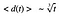Eq. Walk2

For a D-dimensional random walk the argument can be extended to prove that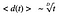Eq. Walk3

But this is wrong! A random walk on average grows as sqrt(t) in any dimension. And we knew this at the time! It was a combination of trusting authority and wanting a simple proof for something we knew to be true that led us — all well-versed in STEM- to accept this fallacious argument.

As an aside, how do we prove that a random walk in any dimension grows as sqrt(t)? With a little bit of simple trigonometry, Pitagoras’ theorem and a sprinkle of recursion. First the trig: How do you add two vectors?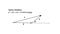Fig. Walk2: Vector addition

Any two vectors in any number of dimensions define a plane. is the angle between them in that plane. Good old Trig. tells us that c^2=a^2+b^2+2ab*cos(phi).

Let ‘a’ be the displacement vector after ‘t’ steps, ‘b’ the ‘t+1’th vector step and ‘c’ the displacement vector after ‘t+1’ steps. To prove what happens on average, all we have to do is take the average of the above equation, keeping in mind that the azimuthal angle

is the random variable. (In higher dimensions, you would also average over multiple polar angles, since strictly speaking the ‘t+1’th vector is uniformly distributed over the sphere, not over phi, but it all works out since it is never the case that the distribution depends on phi.)

Now, a little bit of magic, the angle of the average next step is 90 degrees. Am I saying that the average of [0,360) is 90? No, what I am saying is that the average of cos(phi), with phi ranging between 0 and 360, is zero, and that means the random average direction of the next step is 90 degrees. Simplifying the figure and applying it to the random walk,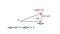Fig. Walk3: On average, each subsequent step is perpendicular to the current displacement vector.

Where <d(t)> as before is the average displacement after ‘t’ steps and the magnitude of the ‘t+1’th step is 1.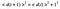Eq. Walk4: Random walk Recursion relation

Which is a recursion relation solved by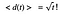Eq. Walk5: Average growth of a random walk in any dimension

# The Shell Theorem

The Shell Theorem states that inside a spherical shell of uniform density, the gravitational force on a test mass ‘m’ is 0. The Wikipedia article reproduces a straightforward, correct but lengthy integral calculus based proof.

However, as I recall it, my college Physics text book in the early 1980s, an edition of Halliday and Resnick back when it was still two volumes, has another, simpler argument.

# The simple proof

This simple argument is present on a NASA K-12 STEM page as well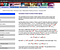Fig. Shell 1: A page from NASA K12 education

and I’ll spell it out with pictures in what follows.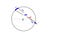Fig. Shell2: A spherical shell of uniform density centered at O, with a test mass ‘m’ in its interior. B and C are parts of the shell in opposite directions from ‘m’, both project the same solid angle at ‘m’.

The circle above represents the spherical shell of uniform density centered at ‘O’ with a point mass ‘m’ somewhere in its interior. Consider a double-cone of infinitesimal solid angle d Omega that intersects the shell at C and B, at distances r_C and r_B respectively. The outward directed gravitational forces on ‘m’ due to the masses of the parts of the shell at B and C are anti-collinear, directed towards the two parts of the shell respectively. The magnitude of the gravitational force on ‘m’ due to B is proportional to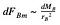Eq. Shell1: The infinitesimal force on m due to B

(‘d’ simply represents the infinitesimal), where the mass of B is the product of the density of the shell times the area of B.

Now, the area of B is simply the square of the distance from ‘m’ times the solid angle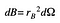Eq. Shell2: The area of the shell part B

(“by definition of the solid angle” on the NASA page), so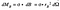Eq. Shell3: The mass of the shell part B

This implies that the force due to B on ‘m’ is proportional to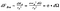Eq. Shell4: The force on m due to B

which is independent of the location of B relative to m. Hence the forces on ‘m’ due to B and C are equal and opposite, and the net force on ‘m’ due to B and C is 0. This applies to all pairs of parts of the shell intersected by the double cone, so you can integrate 0 over half the total solid angle.

Thus we’ve proved that the net gravitational force on a mass in the interior of a uniform shell is 0.

Q.E.D.

Right?

Wrong. See if you can spot the error. It is hard -because we know the Shell Theorem has been proven, by no less than Gauss and Newton and whoever constructed the integral proof- to make ourselves suspicious of yet another proof presented above, specially when it is so simple.

Newton’s proof is of course correct (I mean, how can the great man be wrong? Sorry, I’m being facetious here, that is argumentum ad verecundiam.) and the above “proof” is an incorrect simplification thereof.

In order to see where the above proof goes wrong, I’ll spell out and simplify Newton’s proof. Let’s do some simple 2D trigonometry first.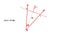Fig. Shell3: The length of an infinitesimal arc

In the above figure, the “observation point” ‘O’ lies on the perpendicular bisector of the infinitesimal segment UV at a distance R from it and the angle projected by UV at O is d theta. So the length of the infinitesimal line segment UV is given by |UV| = R*d theta. Good?

But what happens if the Observation point does not lie along the perpendicular bisector of the line segment?

To start with, in the figure below,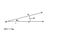Fig. Shell4: The length of another infinitesimal arc

where the line of sight to ‘m’ is again the perpendicular bisector of ST, we have |ST|=r*d omega.

Let’s put those two figures together.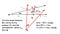Fig. Shell5

It should be flagrantly clear that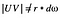Eq. Shell5

Focus on the two triangles defined by SUX and TVX. They are right angled triangles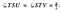Eq. Shell6

and the angle at the apex is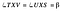Eq. Shell 7

From the definitions of the trigonometric angles,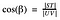Eq. Shell 8: The ratio of the projection of the line segment to its length

Ignoring the existence of O, knowing only the angle between the line of sight from ‘m’ and the perpendicular bisector of UV, this means that the length of the intersection of the plane defined by UV with the perspective from ‘m’ is given by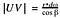Eq. Shell 9: Length of the line segment UV

How does this apply to the argument above? Area elements -like infinitesimal parts (e.g. UV) of a contour- are vectors (*) and have both magnitude and direction. Looking at Fig. Shell1, the normal to the area B is not parallel to the line of sight from ‘m’, it is in fact parallel to the radial vector from ‘O’. So the fallacy in the above argument lies in the claim that

“Now, the area of B is simply the square of the distance from ‘m’ times the solid angle (“by definition of the solid angle” on the NASA page)”

In fact, by definition of the solid angle, only the component of the area of B perpendicular to the line of sight from ‘m’ is the square of the distance from ‘m’ times the solid angle, so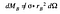Eq. Shell10: The incorrect mass of the part B

So how did a fallacious argument lead to a correct outcome (the Shell Theorem)? Because this is a case where two wrongs happen to make a right. From the trigonometry result above,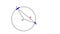Fig. Shell6

the perpendicular component of the area of B perpendicular to the line of sight from ‘m’ is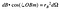Eq. Shell11: The correct area of B

The mass of the element B is then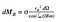Eq. Shell12: The correct mass of B

This implies that the force due to B on ‘m’ is proportional to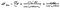Eq. Shell13: The correct gravitational force due to B on m

which is not independent of the location of B but is independent of r_B, the distance between m and B.

How do we proceed? Let’s look at the magnitude of the force due to C on ‘m’. This is proportional to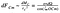Eq. Shell14: The magnitude of the gravitational force due to C on m

Since OCBis an isosceles triangle,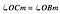Eq. Shell15

and the forces on ‘m’ due to B and C are equal in magnitude. Since they are in opposite directions, the net force on ‘m’ due to B and C is 0. This applies to all pairs of parts of the shell intersected by the double cone, so you can integrate 0 over half the total solid angle.

We’ve now correctly reproduced Newton’s proof that the net gravitational force on a mass in the interior of a uniform shell is 0.

Q.E.D.

# In Conclusion

I have given two examples of a kind of confirmation bias different from the usual one we encounter, the one towards selecting evidence in favor of our pre-existing beliefs. This “new” confirmation bias is a bias towards fallacious arguments that support our (possibly true) pre-existing beliefs. I believe (without examples or proof or anything) that this kind of confirmation bias is more dangerous than the evidence confirmation bias: it is more subtle to detect and it builds pathways in our mind that predispose us to fallacious arguments which can then extend to issues whose truth we should be skeptical about.

If I break it down, it looks a bit like the following, where A is a proposition about the correctness of a logical argument, and B and C are truth-value propositions about facts in different contexts. B is known to be true (but not because of A!), C’s truth value is to be established.

To make the following propositional calculus concrete, let’s use the Random Walk example:

A: is the proposition that the “area of the interior of the mean circle increases linearly in time” argument is correct

B: The mean distance of a random walk increases as the square root of time. Context: two dimensions, but this is not specified, allowing us to implicitly assume it is context independent.

C: The mean distance of a random walk increases as the Dth root of time. Context: D>2 dimensions.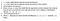Propositional calculus of the dangers of a fallacious argument.

(*) Really an area element is a covector, but just ignore that distinction since we are in Euclidean space. Covectors are cool, and if you understand co-vectors you’ll see that Gradient Descent doesn’t make any sense.

## More from Ranjeet Tate

I stop to miau to cats.